Finding Inverse

Chapter 1 Class 12 Relation and Functions
Concept wise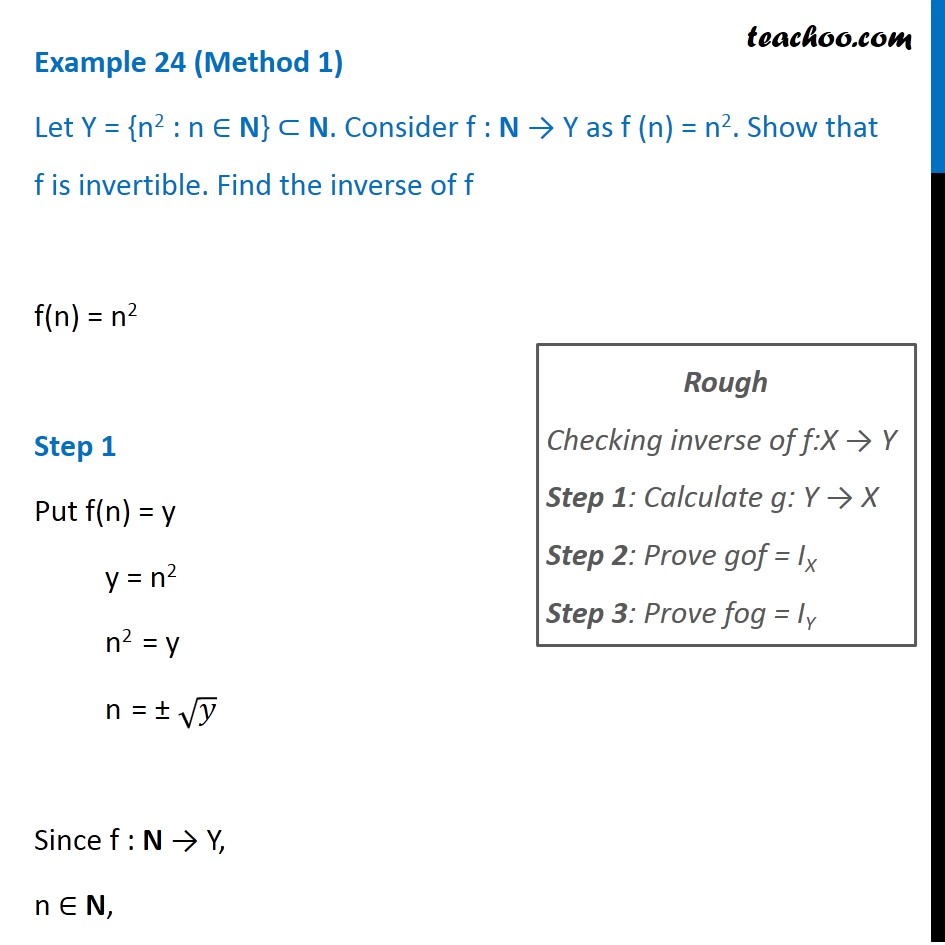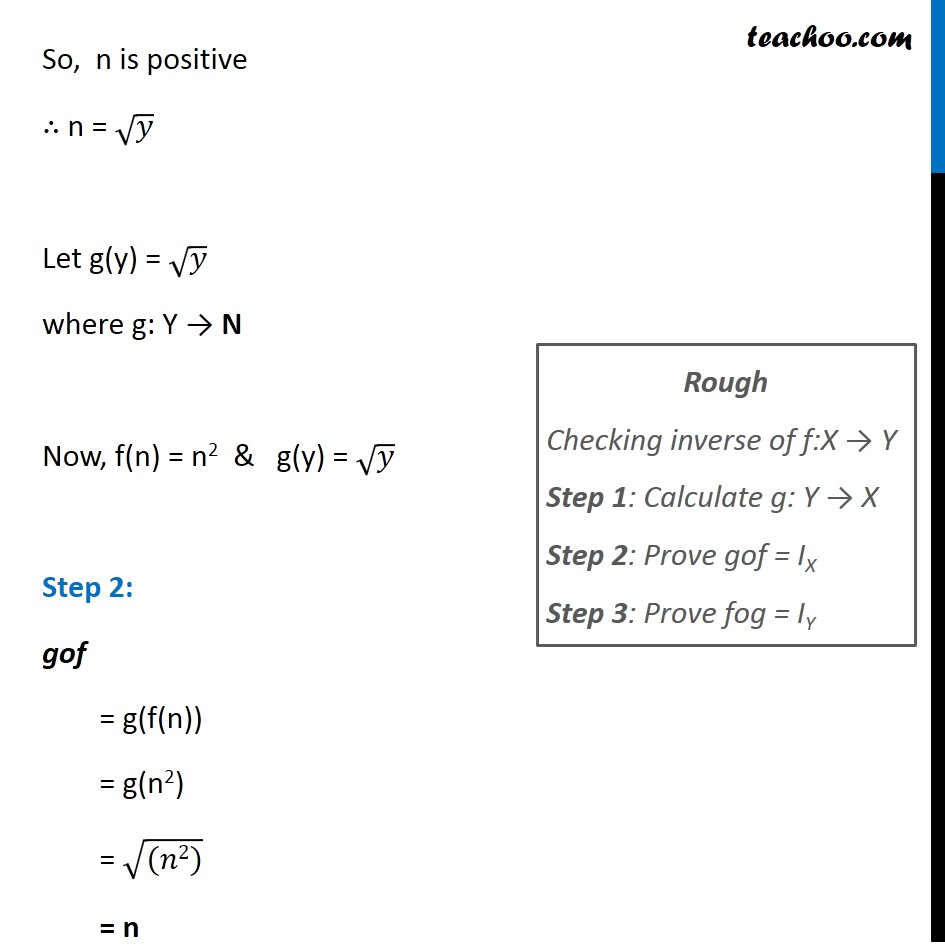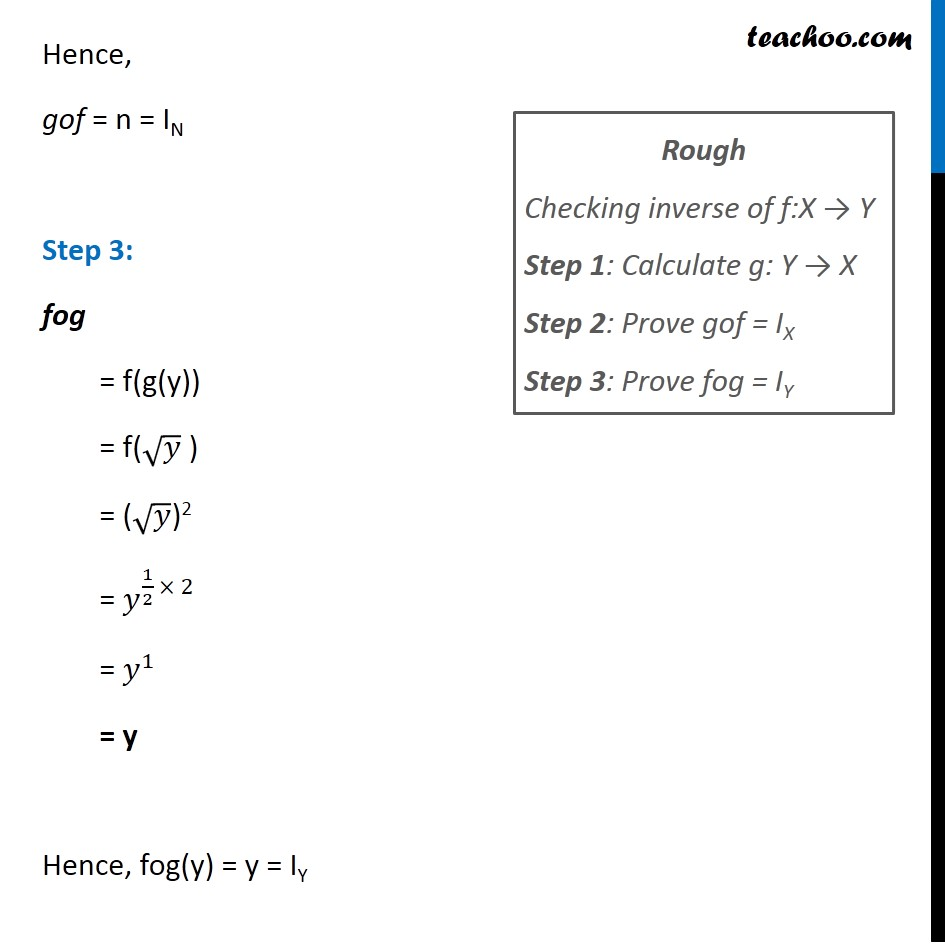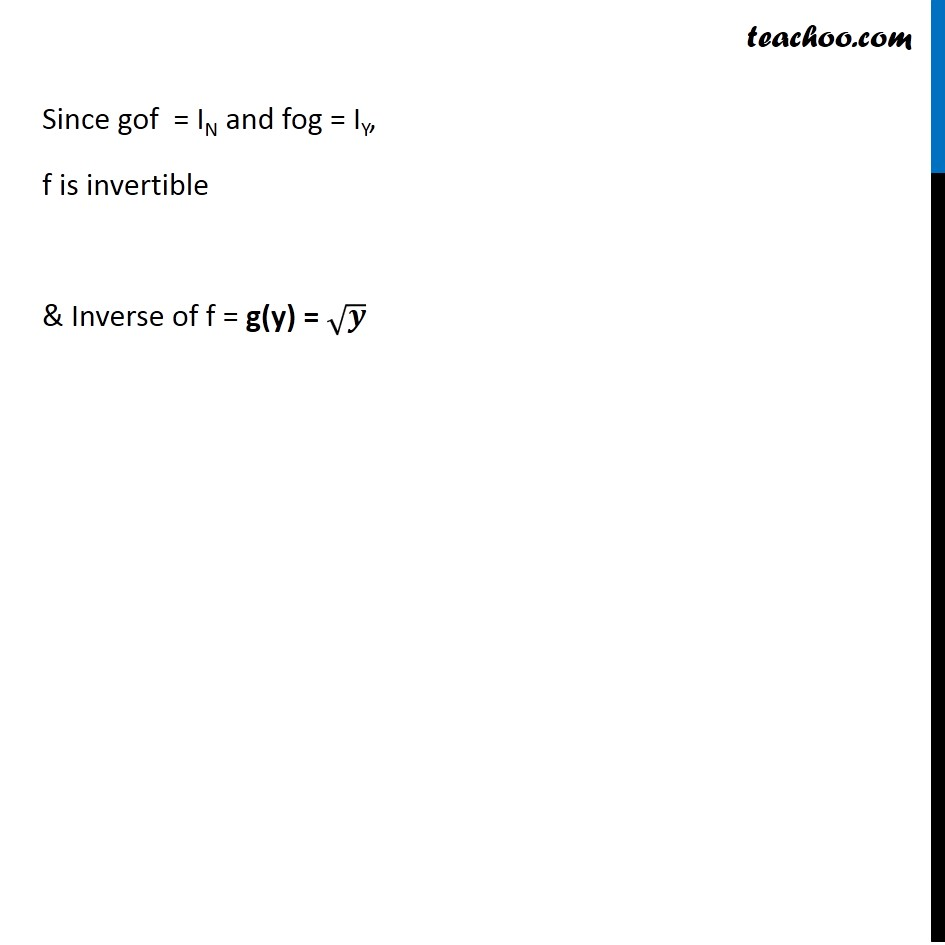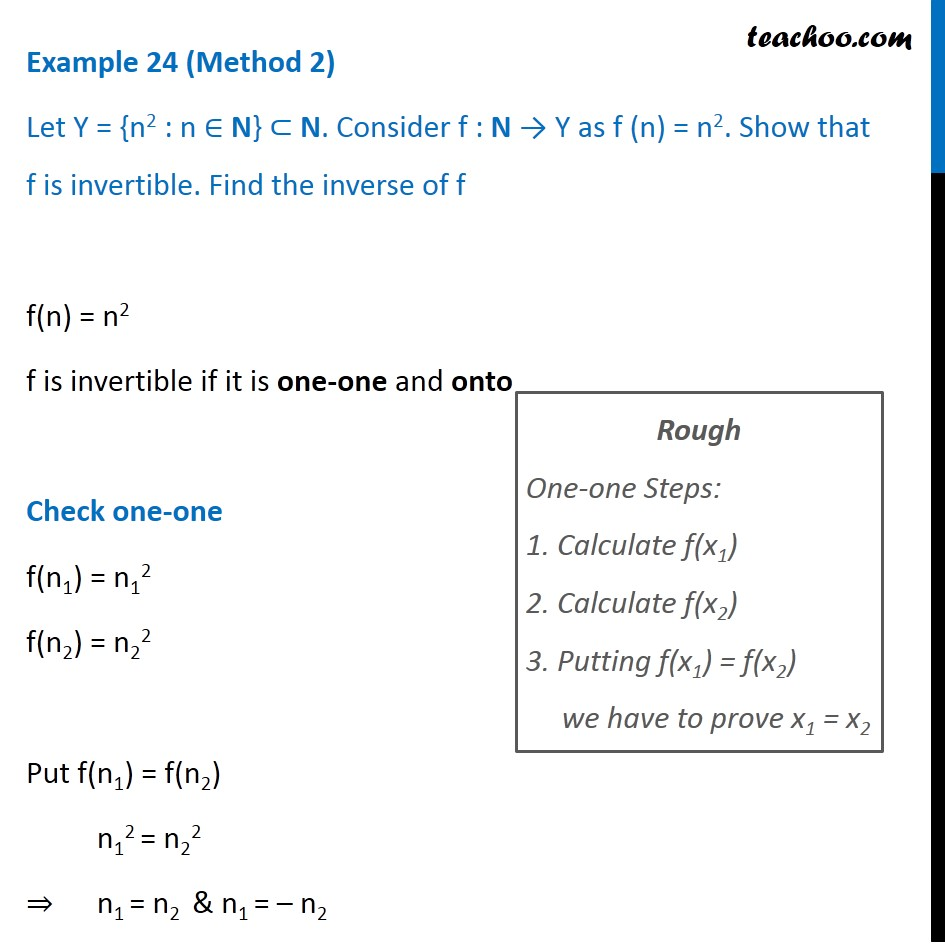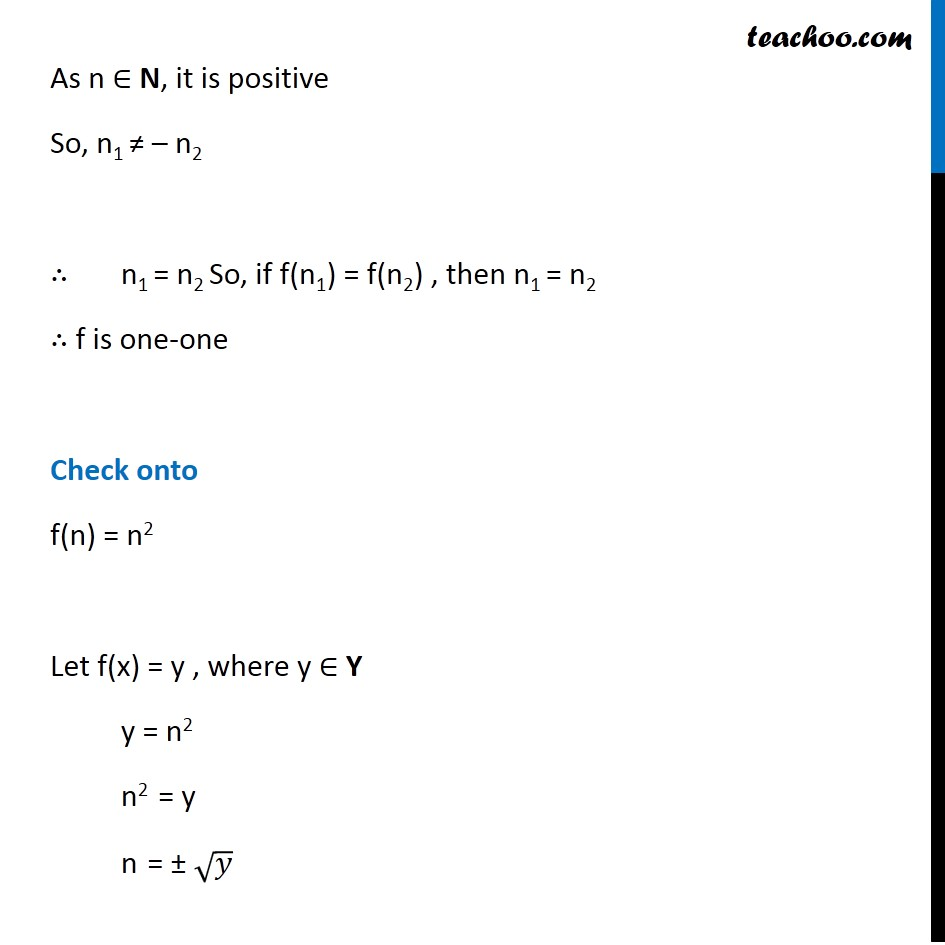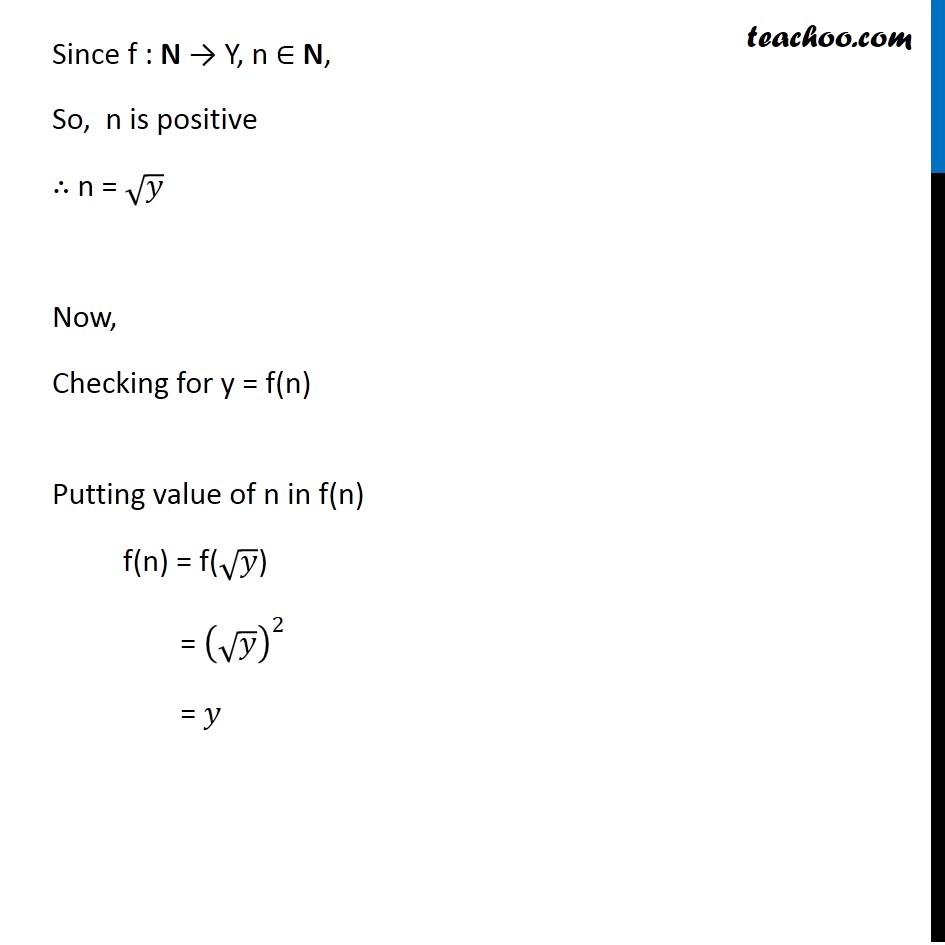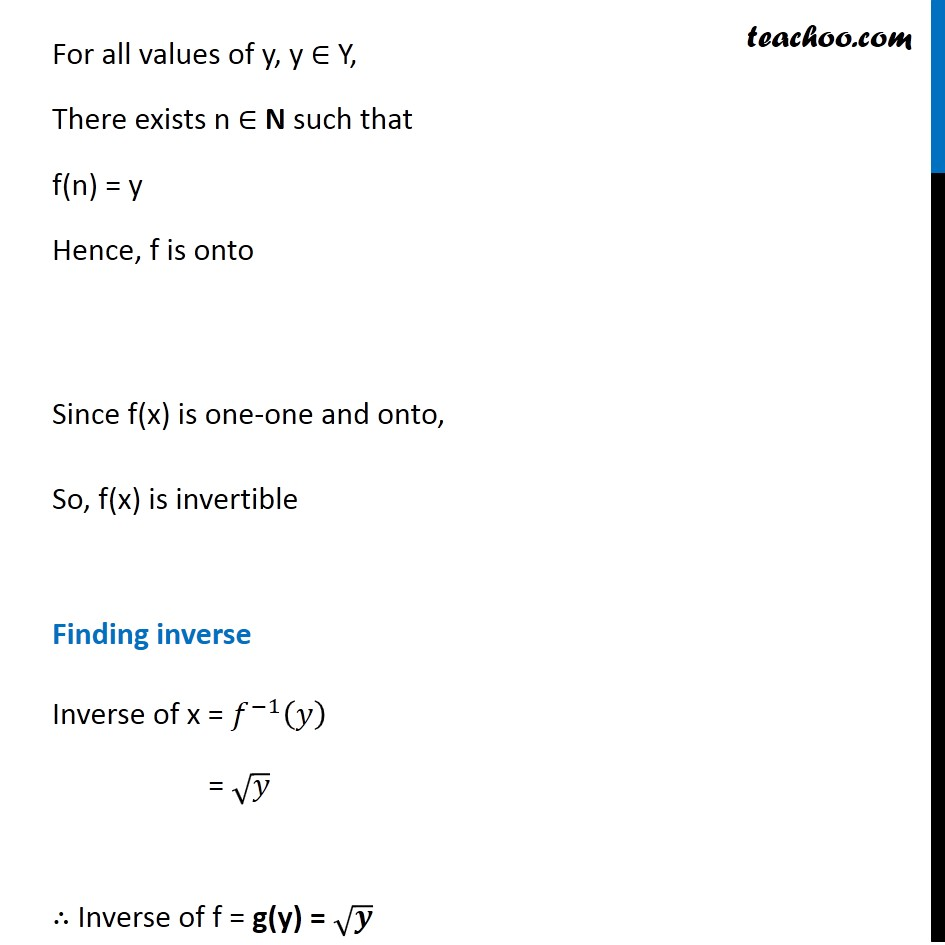Introducing your new favourite teacher - Teachoo Black, at only ₹83 per month

### Transcript

Example 24 (Method 1) Let Y = {n2 : n ∈ N} ⊂ N. Consider f : N → Y as f (n) = n2. Show that f is invertible. Find the inverse of f f(n) = n2 Step 1 Put f(n) = y y = n2 n2 = y n = ± √𝑦 Since f : N → Y, n ∈ N, Rough Checking inverse of f:X → Y Step 1: Calculate g: Y → X Step 2: Prove gof = IX Step 3: Prove fog = IY So, n is positive ∴ n = √𝑦 Let g(y) = √𝑦 where g: Y → N Now, f(n) = n2 & g(y) = √𝑦 Step 2: gof = g(f(n)) = g(n2) = √((𝑛2)) = n Rough Checking inverse of f:X → Y Step 1: Calculate g: Y → X Step 2: Prove gof = IX Step 3: Prove fog = IY Hence, gof = n = IN Step 3: fog = f(g(y)) = f(√𝑦 ) = (√𝑦)2 = 𝑦^(1/2 × 2) = 𝑦^1 = y Hence, fog(y) = y = IY Rough Checking inverse of f:X → Y Step 1: Calculate g: Y → X Step 2: Prove gof = IX Step 3: Prove fog = IY Since gof = IN and fog = IY, f is invertible & Inverse of f = g(y) = √𝒚 Example 24 (Method 2) Let Y = {n2 : n ∈ N} ⊂ N. Consider f : N → Y as f (n) = n2. Show that f is invertible. Find the inverse of f f(n) = n2 f is invertible if it is one-one and onto Check one-one f(n1) = n12 f(n2) = n22 Put f(n1) = f(n2) n12 = n22 ⇒ n1 = n2 & n1 = – n2 Rough One-one Steps: 1. Calculate f(x1) 2. Calculate f(x2) 3. Putting f(x1) = f(x2) we have to prove x1 = x2 As n ∈ N, it is positive So, n1 ≠ – n2 ∴ n1 = n2 So, if f(n1) = f(n2) , then n1 = n2 ∴ f is one-one Check onto f(n) = n2 Let f(x) = y , where y ∈ Y y = n2 n2 = y n = ± √𝑦 Since f : N → Y, n ∈ N, So, n is positive ∴ n = √𝑦 Now, Checking for y = f(n) Putting value of n in f(n) f(n) = f(√𝑦) = (√𝑦)^2 = 𝑦 For all values of y, y ∈ Y, There exists n ∈ N such that f(n) = y Hence, f is onto Since f(x) is one-one and onto, So, f(x) is invertible Finding inverse Inverse of x = 𝑓^(−1) (𝑦) = √𝑦 ∴ Inverse of f = g(y) = √𝒚